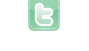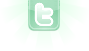## How can I spawn two Objects, in 2 different sizes?

Hey, how do I spawn 2 Objects in 2 different sizes? I have one square which has to be 50x50 and the other one is random between 30 and 50, but I dont know how to give them different sizes.
schniggi wrote...
Hey, how do I spawn 2 Objects in 2 different sizes? I have one square which has to be 50x50 and the other one is random between 30 and 50, but I dont know how to give them different sizes.
Show codes for class Square.
public Squares() { setMyImage(); setMyImage1(); } private void setMyImage() { GreenfootImage img = new GreenfootImage(100,100); img.setColor(randomColor()); img.drawRect(0,0,50,50); setImage(img); } private void setMyImage1() { int x = 30+Greenfoot.getRandomNumber(50)+1; GreenfootImage img = new GreenfootImage(100,100); img.setColor(randomColor()); img.drawRect(0,0,x,x); setImage(img); } public void act() { } private Color randomColor(){ int red = Greenfoot.getRandomNumber(256); int green = Greenfoot.getRandomNumber(256); int blue = Greenfoot.getRandomNumber(256); return new Color(red, green, blue ); }
The constructor will need to know whether to create a fixed sized or a random sized square. A boolean parameter will do the trick:
```public Square(boolean randomSized)
{
if (randomSized) getMyImage1(); else getMyImage();
}```
Then in world use new Square(false) to create a 50x50 square and use new Square(true) to create a random sized one. Your random size is currently set to between 31 and 80. For between 30 and 50, inclusive, use:
`int x = 30+Greenfoot.getRandomNumber(20+1);`
public Squares() { setMyImage(); setMyImage1(); } private void setMyImage() { GreenfootImage img = new GreenfootImage(100,100); img.setColor(randomColor()); img.drawRect(0,0,50,50); setImage(img); } private void setMyImage1() { int x = 30+Greenfoot.getRandomNumber(50)+1; GreenfootImage img = new GreenfootImage(100,100); img.setColor(randomColor()); img.drawRect(0,0,x,x); setImage(img); } public void act() { } private Color randomColor(){ int red = Greenfoot.getRandomNumber(256); int green = Greenfoot.getRandomNumber(256); int blue = Greenfoot.getRandomNumber(256); return new Color(red, green, blue ); } public Squares(boolean randomSized) { if (randomSized) setMyImage1(); else setMyImage(); Is this wrong or false? I get now for both a random Size, but one needs to be a fix size
Nvm, it works